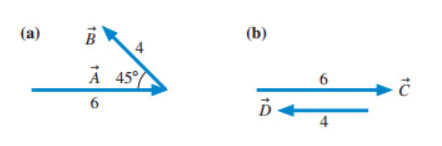# Problem: Evaluate the cross products A×B  and C×D? (Figure 1)Part AWhat is the magnitude of the cross product A×B? Express you answer using two significant figures.Part BWhat is the direction of the cross product A×B? (multiple choice)a) into the plane of the imageb) it is opposite to the direction of Ac) out of the plane of the imaged) A×B is zero vectore) it is opposite to the direction of BPart CWhat is the magnitude of the cross product C×D?Part DWhat is the direction of the cross product C×D? (multiple choice)a) it is opposite to the direction of Cb) C×D is zero vectorc) into the plane of the imaged) it is the opposite to the direction of De) out of the plane of the image

###### FREE Expert Solution

The magnitude of the cross product:

$\overline{){\mathbf{|}}\stackrel{\mathbf{⇀}}{\mathbf{A}}{\mathbf{×}}\stackrel{\mathbf{⇀}}{\mathbf{B}}{\mathbf{|}}{\mathbf{=}}{\mathbf{|}}{\mathbf{A}}{\mathbf{|}}{\mathbf{|}}{\mathbf{B}}{\mathbf{|}}{\mathbf{s}}{\mathbf{i}}{\mathbf{n}}{\mathbf{\theta }}}$

Cross product:

Part A

|A×B| = (6)(4)sin(180°- 45°) = 17

The magnitude of the cross product A×B is 17.

86% (161 ratings)###### Problem Details

Evaluate the cross products A×B  and C×D? (Figure 1)Part A

What is the magnitude of the cross product A×B? Express you answer using two significant figures.

Part B

What is the direction of the cross product A×B? (multiple choice)

a) into the plane of the image
b) it is opposite to the direction of A
c) out of the plane of the image
d) A×B is zero vector
e) it is opposite to the direction of B

Part C

What is the magnitude of the cross product C×D?

Part D

What is the direction of the cross product C×D? (multiple choice)

a) it is opposite to the direction of C
b) C×D is zero vector
c) into the plane of the image
d) it is the opposite to the direction of D
e) out of the plane of the image